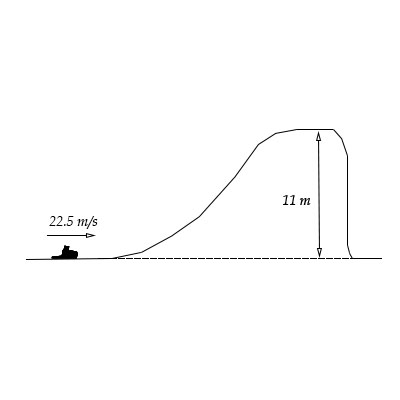# A sled with a rider having a combined mass of 125 \ kg travels over a perfectly smooth icy hill....

## Question:

A sled with a rider having a combined mass of {eq}125 \ kg {/eq} travels over a perfectly smooth icy hill. How far does the sled land from the foot of the cliff?## Kinetic Energy:

The total energy possessed by an object, due to its motion is represented by kinetic energy. It is also defined as the required energy to provide acceleration to a body. When an object is accelerating then, the kinetic energy is mainly gained by that object.

Given data

• The mass of the rider is {eq}m = 125\;{\rm{kg}} {/eq}
• The height is {eq}h = 11\;{\rm{m}} {/eq}
• The speed is {eq}V = 22.5\;{\rm{m/s}} {/eq}

The expression of the conservation of mechanical energy is given by,

{eq}\begin{align*} \dfrac{1}{2}m{V^2} &= \dfrac{1}{2}m{V_1}^2 + mgh\\ \dfrac{1}{2}{V^2} &= \dfrac{1}{2}{V_1}^2 + gh \end{align*} {/eq}

Substitute all the values.

{eq}\begin{align*} \dfrac{1}{2}{\left( {22.5\;{\rm{m/s}}} \right)^2} &= \dfrac{1}{2}{V_1}^2 + \left( {9.81\;{\rm{m/}}{{\rm{s}}^{\rm{2}}}} \right)\left( {11\;{\rm{m}}} \right)\\ \dfrac{1}{2}{V_1}^2 &= \dfrac{1}{2}{\left( {22.5\;{\rm{m/s}}} \right)^2} - \left( {9.81\;{\rm{m/}}{{\rm{s}}^{\rm{2}}}} \right)\left( {11\;{\rm{m}}} \right)\\ {V_1} &= \sqrt {2 \times 145.22\;{{\rm{m}}^{\rm{2}}}{\rm{/}}{{\rm{s}}^{\rm{2}}}} \\ {V_1} &= 17.042\;{\rm{m/s}} \end{align*} {/eq}

The expression of the vertical component of the sleds final velocity is given by,

{eq}\begin{align*} {V_2}^2 &= {V_i} + 2gh\\ {V_2} &= \sqrt {0 + 2gh} \\ {V_2} &= \sqrt {2gh} \end{align*} {/eq}

Substitute all the values.

{eq}\begin{align*} {V_2} &= \sqrt {2\left( {9.81\;{\rm{m/}}{{\rm{s}}^{\rm{2}}}} \right)\left( {11\;{\rm{m}}} \right)} \\ &= 14.69\;{\rm{m/s}} \end{align*} {/eq}

The expression of the vertical component of the sleds final velocity is given by,

{eq}\begin{align*} {V_2} &= {V_i} + gt\\ gt &= {V_2} + 0\\ t &= \dfrac{{{V_2}}}{g} \end{align*} {/eq}

Substitute all the values.

{eq}\begin{align*} t &= \dfrac{{\left( {14.69\;{\rm{m/s}}} \right)}}{{\left( {9.81\;{\rm{m/}}{{\rm{s}}^{\rm{2}}}} \right)}}\\ &= 1.497\;{\rm{s}} \end{align*} {/eq}

The expression of the horizontal distance is given by,

{eq}H = \dfrac{{\left( {{V_1} + {V_1}} \right) \times t}}{2} {/eq}

Substitute all the values.

{eq}\begin{align*} H &= \dfrac{{\left( {17.042\;{\rm{m/s}} + 17.042\;{\rm{m/s}}} \right) \times \left( {1.497\;{\rm{s}}} \right)}}{2}\\ &= 25.51\;{\rm{m}} \end{align*} {/eq}

Thus, the height from which sled land from the foot of the cliff is 25.51 m.